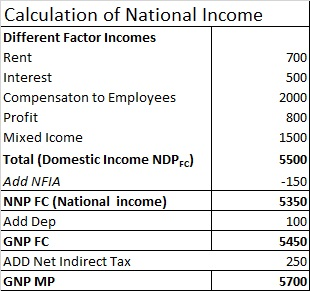Chapter 2 National Income - Part 4 Income Method

Economics Class 12
Macroeconomics

## Calculation of GNP MP by Expenditure Method

Step 1

We calculate National Income (NNP FC)

Step 2

We add Depreciation to get GNP FC

Step 3

We add Net Indirect Tax to arrive at GNP MP

#### Example

Calculate GNP MP With following Data

Net indirect Taxes 200,Depreciaton 100,Profit 20,Rent 10,Mixed Income 30Royalty 50,Interest 30,Compenaton to employees 40,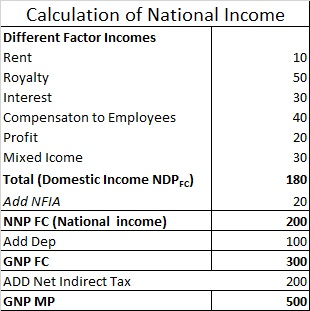#### Example 21.

Calculate GNP at MP from the following data:

 Particulars ₹ in Crores (i) Net indirect tax 900 (ii) Depreciation 400 (iii) Net Factor income from abroad -20 (iv) Rent 1,000 (v) Dividend 500 (vi) Mixed Income 200 (vii) Saving of private corporate sector 400 (viii) Interest 200 (ix) Compensation of employees 100

In this question, we are not given Profit

So we will first calculate Profit

Then we will calculate

Step 1

Calculate Profit

Profit =Dividend +Savings of Corporate Sector + income Tax

Profit = 500 + 400 + 0 = 900

*assume Income tax to be 0

Step 2

Calculate National Income and GNP MP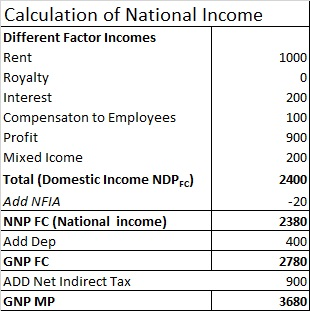#### Question 18

Calculate GNP at MP.

 Particulars ₹  in crores (i) Employee Compensation 600 (ii) Rent and interest 350 (iii) Profit 200 (iv) Indirect Tax 160 (v) Consumption of fixed capital 200 (vi) Mixed Income of the self-employed 850 (vii) Subsidies 40 (viii) Net current transfers  from rest of the world 850 (ix) Net Factor income from abroad (-)100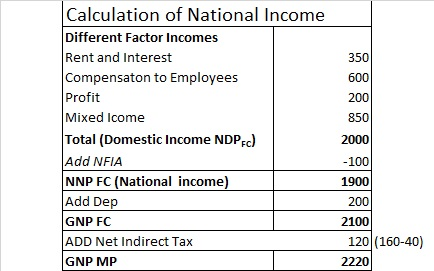#### Question 19.

Calculate GNP at MP.

 Particulars ₹ in crores (i) Indirect tax 200 (ii) Consumption of fixed capital 100 (iii) Factor Income to abroad 250 (iv) Factor Income from abroad 320 (v) Rent 250 (vi) Dividend 220 (vii) Mixed Income 120 (viii) Saving of private corporate sector 200 (ix) Interest 100 (x) Subsidies 200 (xi) Compensation of employees 500 (xii) Corporate tax 400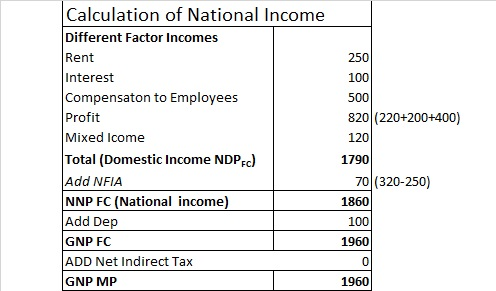#### Question 20.

Calculate "Gross National Product at Market Price" from the following data:

 Particulars ₹  in crores (i) Compensation of employees 2,000 (ii) Interest 500 (iii) Rent 700 (iv) Profits 800 (v)Employers' contribution to social security schemes 201 (vi) Dividends 300 (vii) Consumption of fixed capital 100 (viii) Net indirect taxes 250 (ix) Net exports 70 (x) Net factor income to abroad 150 (xi) Mixed income of self-employed 1,500